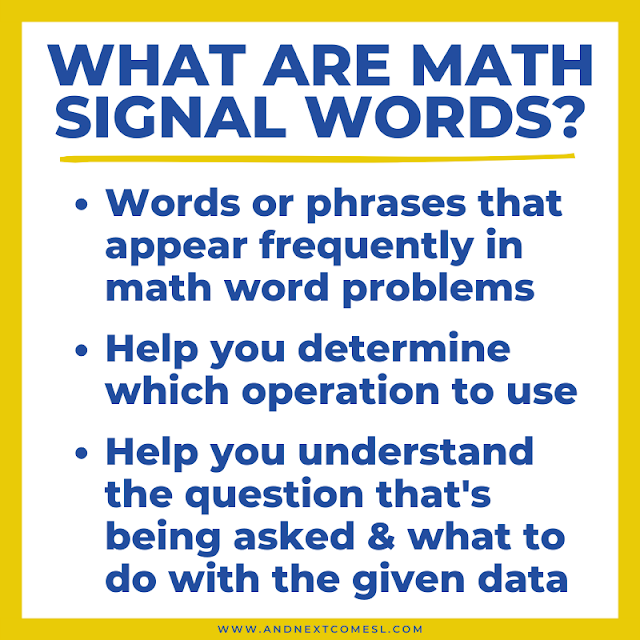# Math Signal Words That Will Improve Comprehension of Word Problems

This blog uses affiliate links. Basically, I make a small commission when you use these links, at no additional cost to you.

Improve comprehension of word problems in math with this free list of math signal words.

The comprehension issues that often accompany hyperlexia can have an impact on other subject areas in school such as math. And, to be honest, I hadn't really considered the impact that these issues might have on something like math word problems. At least, not until I watched this hyperlexia reading comprehension webinar. It really was an aha moment for me.

But it makes sense that word problems in math could be a struggle if comprehension is also an issue.

Just to be clear, we're not talking about simple math sentences here. The ones where they just give some numbers and you have to solve it. No, we're talking about word problems in math. The ones that require a child to read a couple of sentences and then somehow determine what operation to use. Probably those same ones you hated solving as a child yourself.

Now, many kids - not just hyperlexic ones - might read a word problem and have zero clue what it's asking them to do. They can't decipher what the actual question is. So then they have no idea how to begin solving it.

Well, that's where math signal words come in.

Teaching your child about these key words in word problems can significantly boost their understanding of the problem and what's being asked of them. So they're a perfect tool to teach your hyperlexic child about since they can dramatically improve comprehension of these word problems.## So What are Math Signal Words Exactly?

Well, signal words - or key words - are the words or phrases that appear frequently in math word problems.

These specific words or phrases help you determine which operation to use, whether that's addition, subtraction, multiplication, or division.

Basically, they are words that help kids understand the question that is being asked and what to do with the given data in the word problem.## How to Use Signal Words for Math Word Problems

Part of the problem with word problems in math is that a child might not know how to translate the words into an actual math equation to solve. So they get stuck.

Being able to read and understand a word problem is a important skill in math. One that is just as important as knowing how to add or subtract and multiply or divide. However, it's a skill that also requires some good inferencing skills. A skill that many hyperlexic kids might also need some help developing and improving.

But looking for any of the word problem key words listed below can help reduce some of the guesswork for a lot of kids.

So, after reading the word problem, they can search for any important keywords and underline or highlight the ones that they see. Then they can check their list of math signal words (free printable included below!) to determine which operation they should be doing. For example, if a word problem includes the word perimeter, then kids can quickly determine that they need to be adding some numbers together.

Please note that some signal words might indicate more than one operation (e.g., the phrase "in all" could indicate addition or multiplication). So it's important to teach your kids to look for multiple key words in the word problem to help narrow things down.

Now that you know what math signal words are and how to use them, let's take a look at some of the actual key words to watch out for.

You'll see the following words or phrases in addition word problems. They indicate that it's time to add.

• Plus
• And
• Total
• Increase
• Together
• More
• Combine
• In all
• More than
• How many
• How much
• Perimeter

## Subtraction Key Words

When it comes to subtraction word problems, here are some of the more common words you'll see. So when you see any of these words, you'll know it's time to subtract.

• Subtract
• Difference
• Minus
• Take away
• Fewer
• Remain
• Left
• Left Over
• Are not
• Have left
• Less than
• Subtraction
• Decrease
• Less than
• Change
• Exceed
• How many more
• Dropped
• Greater than
• Farther

## Multiplication Key Words

As for multiplication word problems, here's a list of words you might see.

• Times
• Product
• Product of
• Factor
• Multiply
• Multiple
• Double
• Triple
• Groups
• Each
• Area
• Twice
• Total
• By
• Every
• In all
• Multiplied by
• Equal groups

## Division Key Words

And finally we have division word problems. Here are the words to watch out for when it comes to division.

• Quotient
• Divide
• Divided by
• Dividend
• Divisor
• Split
• Share
• Separate
• Parts
• Each
• Equal groups
• Average
• Goes into
• Into
• Ratio
• Distribute
• Every
• Cut up
• Each group has
• Half
• Same
• Shared equally
• Percent
• Out of